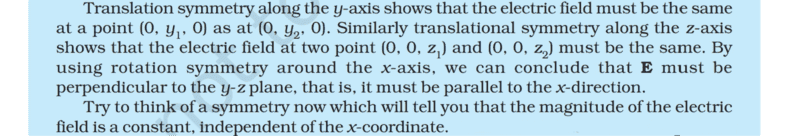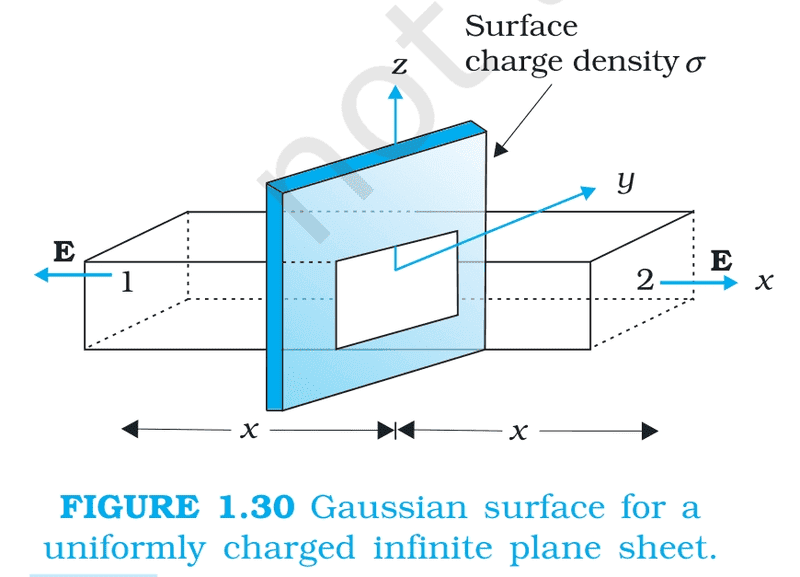# Symmetry & Field of an Infinite uniformly charged plane sheet

Homework Statement:
Think of a symmetry now which will tell you that the magnitude of the electric
field is a constant, independent of the x-coordinate for a infinite uniformly charged plane sheet?
Relevant Equations:
Electric Field of uniformly charged infinite planar sheet = (surface charge density)/ (2× permittivity of free space)
Will translation parallel to x axis work ?
And does symmetry here refer to the symmetry of the sheet which causes the symmetry of the field or something else?

hutchphd
Homework Helper
Think about being on an infinite plane. I look around and everywhere I look is identical. Anywhere I go on the plane there is no preferred direction parallel to the plane. Therefore the field cannot point in any particular parallel direction anywhere so it must point only directly out of the plane everywhere (or be zero). Have you learned Gauss's Law?

•Shreya
Thank you, So here, symmetry means being it (sheet and its field) the same irrespective of position.
But, I am still confused about the field being independent of x coordinate.
This is the whole question. Can you please explain on which symmetry shows field in independent of x coordinate.Think about being on an infinite plane. I look around and everywhere I look is identical. Anywhere I go on the plane there is no preferred direction parallel to the plane. Therefore the field cannot point in any particular parallel direction anywhere so it must point only directly out of the plane everywhere (or be zero). Have you learned Gauss's Law?

Yes, I have learned gauss law and have learnt to derive the field of an infinite plane sheet. This is the image corresponding to the question.